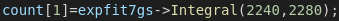# Why there is so large a gap between the experimental data and the integral of fitting function?

I am calculating the integral of the fitting function, but I have found that the integral of fitting function has a large gap compared with the experimental data in the intagral limits. This is the fitting function and the histogram and another plot is the fitting parameters.
The integral of fitting function is 133591; while the sum of experimental data is 16570.

I am sure that the parameters of the function is right, and this is the code I used. The sum of the experimental data is calculate by the histogram:3300+3240+3420+3270+3340=16570You need to take the “bin width” into account, e.g., assuming that the “`histogram`” has “fix bin sizes”:
`function->Integral(xmin, xmax) / histogram->GetBinWidth(histogram->FindFixBin(xmin));`

BTW. As pointed out in another post here, the bins of your histogram are pretty broad, so you should use the “`I`” fit option.

Thank you, I will try it.

—Original—

No, the sum is `3300+3240+3420+3270+3340=16570`, and the integral is when you multiply this sum by the bin width (8 in your case): `(3300+3240+3420+3270+3340)*8= 132560≈133591`

The previous remarks explain the large discrepancy but in general there can be more subtle issues. Normally a fit tries to minimize the distance between the histogram bin height and the value of the fitting function in the center of the bin. The resulting fit function will also deliver the optimal integral as long as the fit function is linear across the bin. In order to make sure that your fit has the correct integral, you should specify the fit option “I”

@yus

``````{
TH1D *h = new TH1D("h", "h", 100, 0., 100.);
for (int i = 1; i <= 100; i++) h->SetBinContent(i, 1.);
cout << "integral (ordinary)         = " << h->Integral() << endl;
cout << "integral (ordinary * width) = " << h->Integral("width") << endl;
h->Rebin(100);
cout << "integral (rebinned)         = " << h->Integral() << endl;
cout << "integral (rebinned * width) = " << h->Integral("width") << endl;
}
``````

This topic was automatically closed 14 days after the last reply. New replies are no longer allowed.